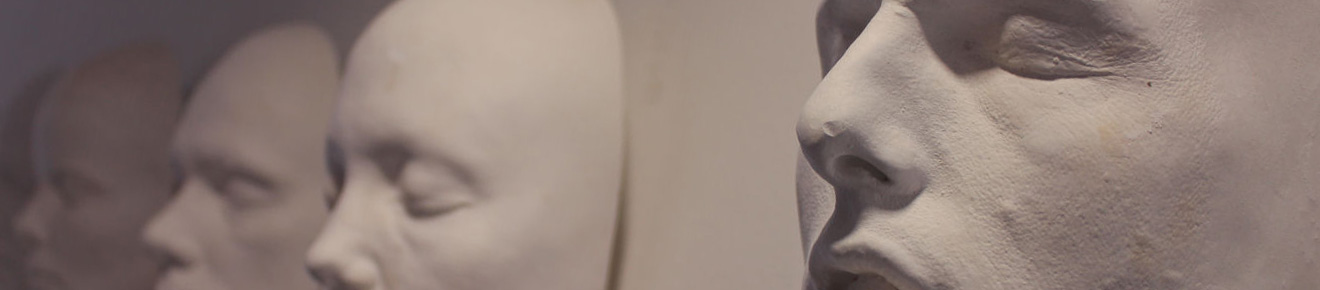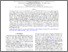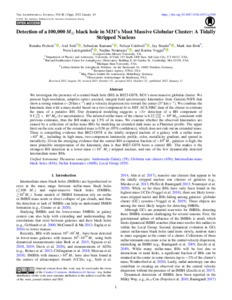# Detection of A ∼100,000 M Black Hole in M31’s Most Massive Globular Cluster: A Tidally Stripped Nucleus

Pechetti, R, Seth, A, Kamann, S, Caldwell, N, Strader, J, Brok, MD, Luetzgendorf, N, Neumayer, N and Voggel, K (2022) Detection of A ∼100,000 M Black Hole in M31’s Most Massive Globular Cluster: A Tidally Stripped Nucleus. The Astrophysical Journal, 924 (2). ISSN 1538-4357Preview
Text
Detection of A ∼100,000 M Black Hole in M31’s Most Massive Globular Cluster A Tidally Stripped Nucleus.pdf - Published Version
We investigate the presence of a central black hole (BH) in B023-G078, M31's most massive globular cluster. We present high-resolution, adaptive-optics assisted, integral-field spectroscopic kinematics from Gemini/NIFS that shows a strong rotation ($\sim$20 km/s) and a velocity dispersion rise towards the center (37 km/s). We combine the kinematic data with a mass model based on a two-component fit to $HST$ ACS/HRC data of the cluster to estimate the mass of a putative BH. Our dynamical modeling suggests a $>$3$\sigma$ detection of a BH component of 9.1$^{+2.6}_{-2.8}\times$10$^4$ M$_\odot$ (1$\sigma$ uncertainties). The inferred stellar mass of the cluster is 6.22$^{+0.03}_{-0.05}\times$10$^6$ M$_\odot$, consistent with previous estimates, thus the BH makes up 1.5% of its mass. We examine whether the observed kinematics are caused by a collection of stellar mass BHs by modeling an extended dark mass as a Plummer profile. The upper limit on the size scale of the extended mass is 0.56 pc (95% confidence), which does not rule out an extended mass. There is compelling evidence that B023-G078 is the tidally stripped nucleus of a galaxy with a stellar mass $>$10$^9$ M$_{\odot}$, including its high mass, two-component luminosity profile, color, metallicity gradient, and spread in metallicity. Given the emerging evidence that the central BH occupation fraction of $>$10$^9$ M$_{\odot}$ galaxies is high, the most plausible interpretation of the kinematic data is that B023-G078 hosts a central BH. This makes it the strongest BH detection in a lower mass ($<$10$^7$ M$_{\odot}$) stripped nucleus, and one of the few dynamically detected intermediate-mass BHs.View Item